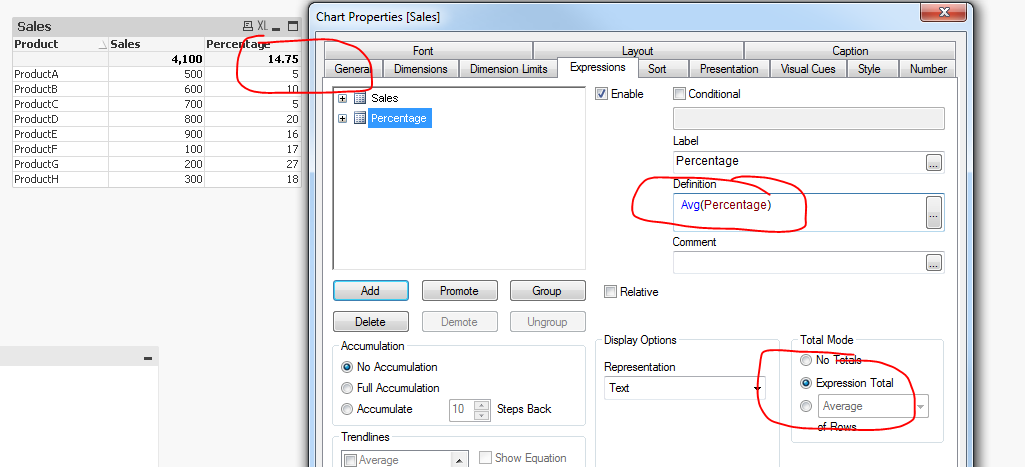Announcements
You can succeed best and quickest by helping others to succeed. Join the conversation.
cancel
Showing results for
Did you mean:Contributor

## Regarding Total Mode as a average in Straight Table

Hi All,

I need to create a dashboard and show sales and percentage as a expression in straight table. Now for totals, percentage has to be displayed as Total Mode = Average.

 Product Sales Percentage 4100 11.8 ProductA 500 5 ProductB 600 10 ProductC 700 5 ProductD 800 20 ProductE 900 16 ProductF 100 17 ProductG 200 27 ProductH 300 18

The total for percentage is displaying 11.8 as shown in the attached files(also above), however if we calculate manually, the percentage total(average) should be 118/8 = 14.75 but it is showing 11.8 since it is calculating it as 118/10(including products for which expression is null).

Please suggest how correct average can be displayed.

Thanks,

Reema

6 RepliesMVP

How about you use this as expression

Avg(Percentage)

with Expression Total as total modeMVP

Use total mode as "Expression total" and use below expression

=avg(Percentage)Contributor
Author

This is actually a dummy data, in actual we need to do sum for expression (Sum(A)/Sum(B) as percentage), is it possible without using the average function in expression?

ThanksMVP

May be try this

Avg(Aggr(Sum(A)/Sum(B), Product))

Still using expression total as total modeContributor
Author

Even this is not working, I will try to create the replica of expression and upload the file for clarity.MVP